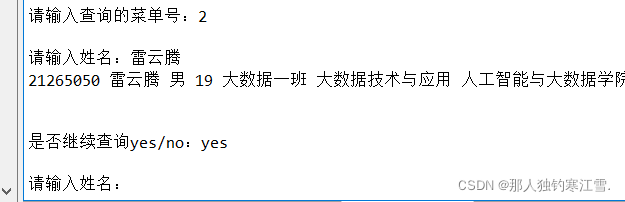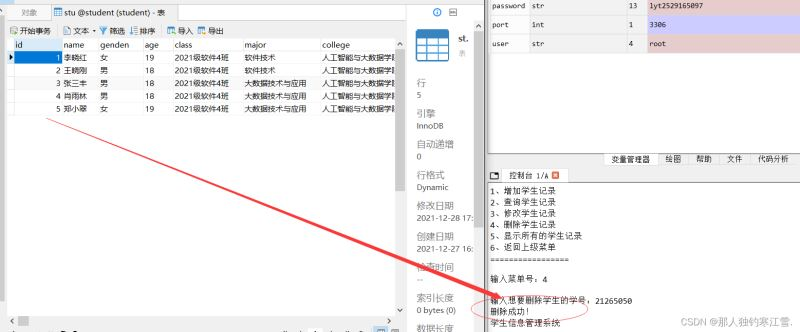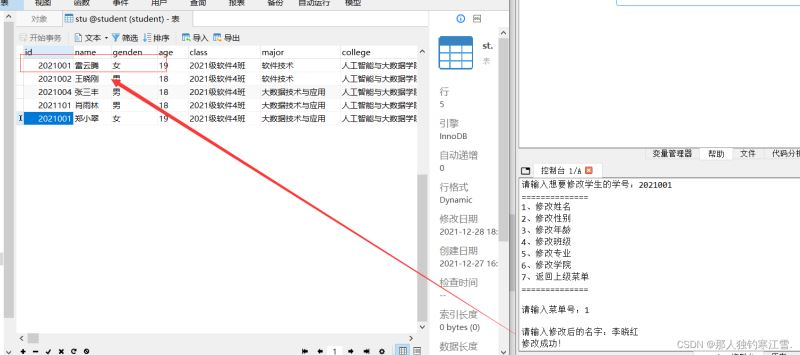﻿ Python通过pymysql调用MySQL进行增删改移查_python_脚本之家
python# Python通过pymysql调用MySQL进行增删改移查

1*)一对一模型

2*)一对多模型

3*)多对多模型

1*)对象模型概念

2*)对象模型特点

## 二、了解关系数据库的概念和特点

i*）关系

ii*）二维表

iii*）记录与字段

VI*）关键字

VII*）外部关键字

### 基本特点

1.关系数据库中的表是二维表，表中的字段必须是不可再分的，即不允许表中表。

2.在同一个表中不允许出现重复的记录。

3.在同一个记录中不允许出现重复的字段。

4.表中记录先后顺序不影响数据的性质，可以交换记录顺序。

5.记录中字段的顺序不影响数据，可以交换字段的顺序。

## 三、常用字段数据类型## 四、使Mysql和Pymysql链接成功```import pymysql

#定义数据库链接参数
host = '127.0.0.1'  #或者用local host
port = 3306
db = 'student'
user = 'root'
def main():
cursor = conn.cursor(pymysql.cursors.SSCursor)  #流式游标,默认返回元组
return cursor

```

1.编写系统登录退出界面

```while True:
print('请选择以下菜单号:')
print('========='*3)
print('1、登录学生信息管理系统')
print('2、退出学生信息管理系统')
print('========='*3)
mc1 = int(input('输入菜单号：'))
if mc1 == 1:
elif mc1 == 2:
print('感谢使用学生信息管理系统！')
break
```

2.编写进入系统用户名和密码

```def login():
```3.编写登录后供选择的菜单

```#登入后的菜单----展开
print("恭喜你成功登录系统!!")
while True:
print('学生信息管理系统')
print('================')
print('1、增加学生记录')
print('2、查询学生记录')
print('3、修改学生记录')
print('4、删除学生记录')
print('5、显示所有的学生记录')
print('6、返回上级菜单')
print('=================')
mc2 = int(input('输入菜单号：'))
if mc2 == 1:
elif mc2 == 2:
query_student()
elif mc2 == 3:
update_student
elif mc2 == 4:
delete_student()
elif mc2==5:
print_student()
else:
break
else:
print('账号或密码错误！')
```

4.编写增加学生记录的程序

```#插入学生记录
cursor = main()
id = int(input('学号：'))
name = input('姓名：')
gender = input('性别：')
age = int(input('年龄：'))
class1 = input('班级：')
major = input('专业：')
college = input('学院：')
add = cursor.execute('insert into stu (id, name, gender, age, class1, major, college)\
values(%s,%s,%s,%s,%s,%s,%s)',(id, name, gender, age, class1, major, college))
conn.commit()
print('插入成功！')
else:
print('插入失败！')
```I*)按照学号查询学生记录

```#按学号查询
def Q_by_id():
cursor = main()
choice_id = int(input('请输入学号：'))
cursor.execute('select * from stu where id =%s',(choice_id))
students = cursor.fetchall()
for stu in students:
print(stu, stu, stu, stu, stu, stu, stu)
print('查询成功')
re = input('是否继续查询(yes/no)：')
if re == 'yes':
Q_by_id()
else:
query_student()
```

II*)按照姓名查询学生记录

```#按姓名查询（以防学号输入错误）
def Q_by_name():
cursor = main()
choose_name = input('请输入姓名：')
cursor.execute('select * from stu where name =%s',(choose_name))
students = cursor.fetchall()
for stu in students:
print(stu, stu, stu, stu, stu, stu, stu)
print()
re = input('是否继续查询yes/no：')
if re == 'yes':
Q_by_name()
else:
query_student()
```

III*)查询所有学生的信息记录

```#查询所有学生
def Q_all():
cursor = main()
cursor.execute('select * from stu')
students = cursor.fetchall()
for student in students:
print('\t{}\t{}\t{}\t{}\t{}\t{}\t{}' .format(student, student, student, student, student, student, student))
```VI*)编写"是否"进行查询记录

``` re = input('是否继续查询(yes/no)：')
if re == 'yes':
Q_by_id()
else:
query_student()
```VII*)写出登录菜单后还要写出可供我们查询的菜单

```#查询的菜单
def query_student():
while True:
print('查询学生记录')
print('================')
print('1、按学号查询学生记录')
print('2、按姓名查询学生记录')
print('3、查询全部学生记录')
print('4、返回上级菜单')
print('=================')
mc3 = int(input('请输入查询的菜单号：'))
if mc3 == 1:
Q_by_id()
elif mc3 == 2:
Q_by_name()
elif mc3 == 3:
Q_all()
else:
break
```VII*)编写删除学生记录程序

```#删除的菜单
def delete1_student():
print('============================')
print('1、删除学生所有信息')
print('2、回到初始界面')
print('============================')
if mc4 == 1:
delete_student()
elif mc4 == 3:
``````def update_student():
cursor = main()
cur= int(input('请输入想要修改学生的学号：'))
cursor.execute('select * from stu where id = %s', (cur))
if cursor.fetchall() == []:
print('未查找到学号是{}的学生'.format(cur))
mc3 = input('是否重新查询？(yes/no)')
if mc3 != 'no':
update_student()
else:
else:
print('==============')
print('1、修改姓名')
print('2、修改性别')
print('3、修改年龄')
print('4、修改班级')
print('5、修改专业')
print('6、修改学院')
print('7、返回上级菜单')
print('==============')
mc2 = int(input('请输入菜单号：'))
if mc2 == 1:
name = input('请输入修改后的名字：')
a = cursor.execute('update stu set name = %s where id = %s', (name, cur))
if a == 1:
conn.commit()
print('修改成功！')
else:
print('修改失败！')
elif mc2 == 2:
gender1 = input('请输入修改后的性别：')
a = cursor.execute('update stu set genden = %s where id = %s', (gender1, cur))
if a > 1:
conn.commit()
print('修改成功！')
else:
print('修改失败！')
elif mc2 == 3:
age1 = int(input('请输入修改后的年龄：'))
a = cursor.execute('update stu set age = %s where id = %s', (age1, cur))
if a > 1:
conn.commit()
print('修改成功！')
else:
print('修改失败！')
elif mc2 == 4:
class1 = input('请输入修改后的班级：')
a = cursor.execute('update stu set class = %s where id = %s', (class1, cur))
if a > 1:
conn.commit()
print('修改成功！')
else:
print('修改失败！')
elif mc2 == 5:
major1 = input('请输入修改后的专业：')
a = cursor.execute('update stu set major = %s where id = %s', (major1, cur))
if a > 1:
conn.commit()
print('修改成功！')
else:
print('修改失败！')
elif mc2 == 6:
college1 = input('请输入修改后的学院：')
a = cursor.execute('update stu set college = %s where id = %s', (college1, cur))
if a > 1:
conn.commit()
print('修改成功！')
else:
print('修改失败！')
else:
pass#占一个空位符
```1.为什么会写代码

2.特定思维

3.思维灵感

4.脑+手